## 4.2Fixed value and ﬁxed gradient

In an equation for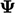that is discretised to form a matrix equation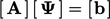, there are two terms that include properties interpolated to faces:

• an advection term of the form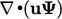which is discretised by Eq. (3.8 ) in extensive form (see Sec. 3.5 ) as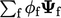;
• a Laplacian term of the form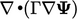which is discretised by Eq. (3.2 ) in extensive form as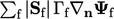.

The advection term requires the value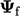and Laplacian term requires the surface normal gradient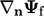at faces. When a face is part of a boundary patch, any gradient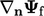and/or value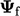must be speciﬁed through boundary conditions.

The ﬁxed value, or Dirichlet condition,1 is the ﬁrst type of boundary condition, where the boundary value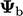is speciﬁed. For example, at an inlet patch, we might specify a temperature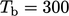K of the ﬂuid ﬂowing into the domain.

The ﬁxed gradient, or Neumann condition,2 is the second type, in which the gradient normal to the boundary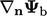is speciﬁed (where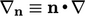). In many cases, the applied normal gradient is zero, which is a common condition applied to many ﬁelds, including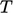, at an outlet patch, i.e. where the ﬂuid ﬂows out of the domain.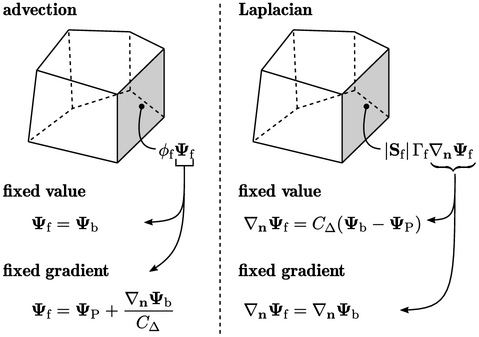When an advection term is discretised, a ﬁxed value condition is applied by substituting the face value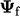with the patch value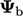, i.e. setting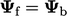. When a ﬁxed gradient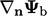is speciﬁed, the face value is expressed as follows, where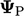is the value in the cell adjacent to each face: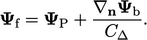(4.2)
When a Laplacian term is discretised, a ﬁxed gradient boundary condition is applied by substituting the face normal gradient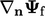with the patch face normal gradient, i.e. setting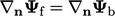. When a ﬁxed valueis speciﬁed, the face normal gradient is expressed by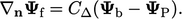(4.3)

1Peter Dirichlet, Über einen neuen Ausdruck zur Bestimmung der Dictigkeit einerunendlich dünnen Kugelschale, wenn der Werth des Potentials derselben in jedem Punkte ihrer Oberﬂäche gegeben ist, 1850.
2after Carl Neumann 1832-1925.

Notes on CFD: General Principles - 4.2 Fixed value and ﬁxed gradient